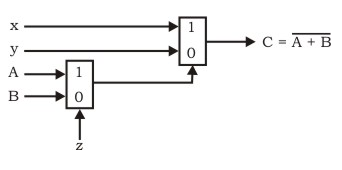# GATE | Gate IT 2005 | Question 48

• Last Updated : 19 Nov, 2018

The circuit shown below implements a 2-input NOR gate using two 2-4 MUX (control signal 1 selects the upper input). What are the values of signals x, y and z?(A) 1, 0, B
(B) 1, 0, A
(C) 0, 1, B
(D) 0, 1, A

Explanation:
In MUX1, equation is : g = Az + Bz’
In MUX2, equation is : f = xg + yg’ = x(Az + Bz’) + y (Az + Bz’)’

Function ‘f’ should be equal to (A + B)’ .

Using hit and try method, put x = 0, y = 1 and z = A.
f = 0(AA + BA’) + 1(AA + BA’)’
f = (A + A’B)’
f = (A + B)’

Thus, option (D) is correct.

Please comment below if you find anything wrong in the above post.

Quiz of this Question

My Personal Notes arrow_drop_up